# Maths Video Lesson 3 Revision eNotes Worksheet and Solution# Contents

Contents 2

3rd Year Worksheet 1 and Solutions 4

Example 1 & Example 2 4

Example 3 4

Example 4 5

Example 5 & Example 6 6

Example 7 & Example 8 7

Example 9 7

Example 10 8

Example 11 8

All revision notes have been produced by mockness ltd for iRevise.com.

Email: info@iRevise.com

All rights reserved; no part of this publication may be reproduced, stored in a retrieval system or transmitted, in any form or by any means, electronic, mechanical, photocopying, recording, reprinting, or otherwise without either the prior written permission of IRevise.com or a license permitting copying in the Republic of Ireland and United Kingdom issued by the copyright licensing Agency.

# 3rd Year Worksheet 1 and Solutions

Now at this stage you should have watched the free Lesson 1 maths tutorial on solving quadratics and read the iRevise.com lesson 1 revision notes. We are now bringing you a worksheet and solutions.

## Example 1 Example 2

Solve 4y² 1 = 0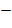(2y)² (1)² =0(2y+1)(2y-1) =0

2y+1=0 Or 2y-1=0

Y= Y=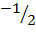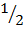Solve 2x² + x 3 = 0 3= 1.3(2x+3)(x1) =0 = 1.3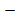2x+3=0 Or x1=02x=3 x=1X=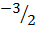## Example 3

Solve

(2y+3)(y+1) = (y+3)²

2y²+ 2y + 3y + 3 = y²+ 6y + 9

2y² + 5y+ 3 y² 6y 9 = 0y² y 6 = 0(y3)(y+2) = 0y3 = 0 Or y+2=0y=3 y=2

## Example 4

Solve,

3x² + 5x – 12 = 0 12(3x-4)(x+3)=0 = -1.12

3x-4=0 Or x+3= 0 = 1.123x=4 x=3 = 2.6X= =2. 6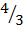= 3.4= 3. 4Or

A= 3 B=5 C=-12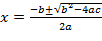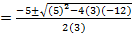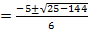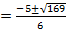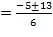Or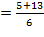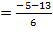= 0r ...